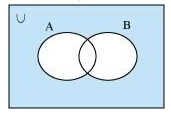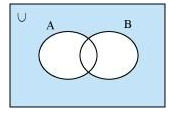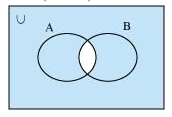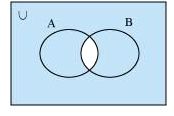# NCERT Solutions For Class 11 Maths Chapter 1 Exercise 1.5

## Chapter 1 Ex.1.5 Question 1

Let $$U = \left\{ {1,2,3,4,5,6,7,8,9} \right\},\;A = \left\{ {1,2,3,4} \right\},\;B = \left\{ {2,4,6,8} \right\}$$ and $$C = \left\{ {3,4,5,6} \right\}$$. Find

(i) $$A^\prime$$

(ii) $${B}^\prime$$

(iii) $$\left( {A \cup C} \right)^\prime$$

(iv)$$\left( {A \cup B} \right)^\prime$$

(v) $$\left( {A\prime } \right)\prime$$

(vi) $$\left( {B-C} \right)^\prime$$

### Solution

$$U = \left\{1,2,3,4,5,6,7,8,9\right\},\;A = \left\{ 1,2,3,4 \right\},\;B = \left\{ {2,4,6,8} \right\}$$ and $$C = \left\{ 3,4,5,6 \right\}$$

(i) $$A\prime = \left\{ {5,6,7,8,9} \right\}$$

(ii) $$B\prime = \left\{ {1,3,5,7,9} \right\}$$

(iii) $$A \cup C = \left\{ {1,2,3,4,5,6} \right\}$$

Therefore, $$\left( {A \cup C} \right)\prime = \left\{ {7,8,9} \right\}$$

(iv) $$A \cup B = \left\{ {1,2,3,4,6,8} \right\}$$

Therefore, $$\left( {A \cup C} \right)\prime = \left\{ {7,8,9} \right\}$$

(v)$$(A^\prime)^\prime=A=\left\{ {1,2,3,4} \right\}$$

(vi) $$B-C = \left\{ {2,8} \right\}$$

Therefore, $$\left( {B-C} \right)\prime = \left\{ {1,3,4,5,6,7,9} \right\}$$

## Chapter 1 Ex.1.5 Question 2

If $$U = \left\{ {a,b,c,d,e,f,g,h} \right\}$$ , find the complements of the following sets:

(i) $$A = \left\{ a,b,c \right\}$$

(ii) $$B = \left\{ d,e,f,g \right\}$$

(iii) $$C = \left\{a,c,e,g \right\}$$

(iv) $$D = \left\{f,g,h,a\right\}$$

### Solution

(i) $${A}^\prime = \left\{ d,e,f,g,h \right\}$$

(ii) $${B}^\prime = \left\{ a,b,c,h \right\}$$

(iii) $${C}^\prime = \left\{ b,d,f,h \right\}$$

(iv) $${D}^\prime = \left\{ b,c,d,e \right\}$$

## Chapter 1 Ex.1.5 Question 3

Taking the set of natural numbers as the universal set, write down the complements of the following sets:

(i) {$$x:x$$ is an even natural number}

(ii) {$$x:x$$ is an odd natural number}

(iii) {$$x:x$$ is a positive multiple of $$3$$}

(iv) {$$x:x$$ is a prime number}

(v) {$$x:x$$ is a natural number divisible by $$3$$ and $$5$$}

(vi) {$$x:x$$ is a perfect square}

(vii) {$$x:x$$ is perfect cube}

(viii) $$\left\{x:x + 5 = 8\right\}$$

(ix) $$\left\{x:2x + 5 = 9 \right\}$$

(x) $$\left\{ x:x \ge 7 \right\}$$

(xi) $$\left\{ {x:x \in N\;{\rm{and}}\;2x + 1 > 10} \right\}$$

### Solution

$$U = N$$: Set of natural numbers

(i) {$$x:x$$ is an even natural number} ´ $$=$$ {$$x:x$$ is an odd natural number}

(ii) {$$x:x$$ is an odd natural number} ´ $$=$$ {$$x:x$$ is an even natural number}

(iii) {$$x:x$$ is a positive multiple of $$3$$} ´ $$=$$ {$$x:x$$ $$∈ N$$ and $$x$$ is not a multiple of $$3$$}

(iv) {$$x:x$$ is a prime number} ´ $$=$${ $$x:x$$ is a positive composite number and $$x~=1$$}

(v) {$$x:x$$ is a natural number divisible by $$3$$ and $$5$$} ´ $$=$$ {$$x:x$$ is a natural number that is not divisible by $$3$$ or $$5$$}

(vi) {$$x:x$$ is a perfect square}´ $$=$$ { $${x:x} \in N$$ and $$x$$ is not a perfect square}

(vii) {$$x:x$$ is a perfect cube}´ $$=$$ {$$x:x \in N$$ and $$x$$ is not a perfect cube}

(viii) $${\left\{ {x:x + 5 = 8} \right\}^\prime } = \left\{ {x:x \in N\;{\rm{and}}\;x \ne 3} \right\}$$

(ix) $${\left\{ {x:2x + 5 = 9} \right\}^\prime } = \left\{ {x:x \in N\;{\rm{and}}\;x \ne 2} \right\}$$

(x) $${\left\{ {x:x \ge 7} \right\}^\prime } = \left\{ {x:x \in N\;{\rm{and}}\;x < 7} \right\}$$

(xi) {$$x:x ∈ N$$ and $$2x+1>10$$ }$$^\prime=$$ $$\left\{ {x:x ∈ N \text{and} \,x<\frac{9}{2} } \right\}$$

## Chapter 1 Ex.1.5 Question 4

If $$U = \left\{ {1,2,3,4,5,6,7,8,9} \right\},\;A = \left\{ {2,4,6,8} \right\}$$ and $$B=\left\{ 2,3,5,7 \right\}$$. Verify that

(i)$${\left( {A \cup B} \right)^\prime } = A' \cap B'$$

(ii) $${{\left( A\cap B \right)}^{\prime }}={A}'\cup {B}'$$

### Solution

(i) $$U = \left\{ {1,2,3,4,5,6,7,8,9} \right\},\;A = \left\{ {2,4,6,8} \right\}$$ and $$B = \left\{ {2,3,5,7} \right\}$$

\begin{align}A \cup B&= \left\{ {2,3,4,5,6,7,8} \right\}\\{\left( {A \cup B} \right)^\prime }&= \left\{ {1,9} \right\}\\A'&= \left\{ {1,3,5,7,9} \right\}\\B'&= \left\{ {1,4,6,8,9} \right\}\\A' \cap B'&= \left\{ {1,9} \right\}\\{\left( {A \cup B} \right)^\prime } &= A' \cap B'\end{align}

(ii) $$U = \left\{ {1,2,3,4,5,6,7,8,9} \right\},\;A = \left\{ {2,4,6,8} \right\}$$ and $$B = \left\{ {2,3,5,7} \right\}$$

\begin{align}A \cap B&= \left\{ 2 \right\}\\{\left( {A \cap B} \right)^\prime } &= \left\{ {1,3,4,5,6,7,8,9} \right\}\\A' \cup B' &= \left\{ {1,3,4,5,6,7,8,9} \right\}\\{\left( {A \cap B} \right)^\prime } &= A' \cup B'\end{align}

## Chapter 1 Ex.1.5 Question 5

Draw appropriate Venn diagram for each of the following:

(i) $${\left( {A \cup B} \right)^\prime }$$

(ii) $$A' \cap B'$$

(iii) $${\left( {A \cap B} \right)^\prime }$$

(iv) $$A' \cup B'$$

### Solution

(i) $${\left( {A \cup B} \right)^\prime }$$(ii) $$A' \cap B'$$(iii) $${\left( {A \cap B} \right)^\prime }$$(iv) $$A' \cup B'$$## Chapter 1 Ex.1.5 Question 6

Let $$U$$ be the set of all triangles in a plane. If $$A$$ is the set of all triangles with at least one angle different from $$60^\circ$$, what is $$A$$′?

### Solution

$$A′$$ is the set of all equilateral triangles.

## Chapter 1 Ex.1.5 Question 7

Fill in the blanks to make each of the following a true statement:

(i) $$A \cup A' =\underline{\;\;\;\;}$$

(ii) $$\phi \prime \cap A =\underline{\;\;\;\;}$$

(iii) $$A \cap A' =\underline{\;\;\;\;}$$

(iv) $$U' \cap A =\underline{\;\;\;\;}$$

### Solution

(i) $$A \cup A' = \underline{\;U\;}$$

(ii) $$\phi \prime \cap A = \underline{\;U \cap A = A\; }$$

(iii) $$A \cap A' = \underline{\;\phi\;}$$

(iv) $$U' \cap A = \underline{\;\phi \cap A = \;\phi \;}$$

Instant doubt clearing with Cuemath Advanced Math Program